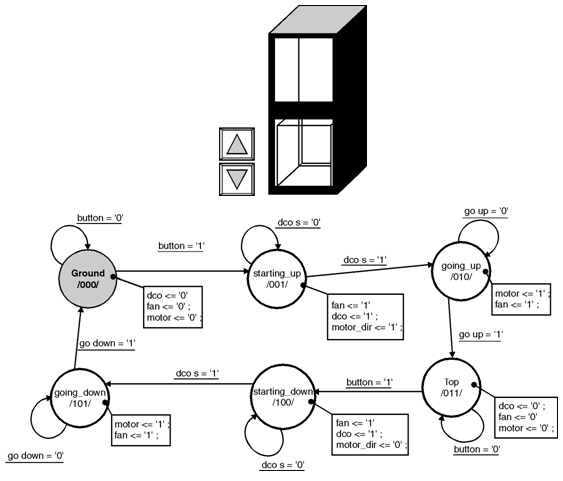Home > finite state machines > FSM Applications > Lift Controller

A Lift Controller

Assumptions :

• Lift service is between two floors only.
• Lift doors closed /opened automatically.
• Lift fan switch on/off automatically (used only when lift is in use).
• If lift doors remain open due to malfunctioning of door etc. lift will not start.
• The direction of motion of motor changed automatically according to position of lift.
• No lift calling system.

Lift controller operation :

• If the lift is downstairs,
1. Close the doors
2. Start the lift fan and check for closed door.
3. Select the motor direction to upward
4. Start motor and go up.
5. Check for top floor position
6. Switch off lift fan and motor
7. Open the doors
• If the lift is upstairs,
1. Close the doors
2. Start the lift fan and check for closed door.
3. Select the motor direction to downward
4. Start motor and go down.
5. Check for ground floor position
6. Switch off lift fan and motor
7. Open the doors
Table below shows the input and output signals opeartion.

State diagram :VHDL code for lift controller :

library IEEE;
use IEEE.std_logic_1164.all;
use IEEE.std_logic_arith.all;
use IEEE.std_logic_unsigned.all;
entity lift_ctr is
port (    button: in STD_LOGIC;
clk: in STD_LOGIC;
dco_s: in STD_LOGIC;
go_down: in STD_LOGIC;
go_up: in STD_LOGIC;
dco: out STD_LOGIC;
fan: out STD_LOGIC;
motor: out STD_LOGIC;
motor_dir: out STD_LOGIC);
end lift_ctr;
architecture lift_ctr_arch of lift_ctr is
attribute enum_encoding: string;
type State_type is  (Ground, starting_up, going_up,  Top,  starting_down,
going_down );
attribute enum_encoding of State_type: type is
"000 " &		-- Ground
"001 " &		-- starting_up
"010 " &		-- going_up
"011 " &		-- Top
"100 " &		-- starting_down
"101" ; 		-- going_down
signal State: State_type;
begin
State_machine: process (clk)
begin
if clk'event and clk = '1' thbbben
case State is
when Ground =>
dco <= '0';
fan <= '0';
motor <= '0';
if button = '1' then
State <= starting_up;
elsif button = '0' then
State <= Ground;
end if;
when starting_up =>
fan <= '1';
dco <= '1';
motor_dir <= '1';
if dco_s = '1' then
State <= going_up;
elsif dco_s = '0' then
State <= starting_up;
end if;
when going_up =>
motor <= '1';
fan <= '1';
if go_up = '1' then
State <= Top;
elsif go_up = '0' then
State <= going_up;
end if;
when Top =>
dco <= '0';
fan <= '0';
motor <= '0';
if button = '1' then
State <= starting_down;
elsif button = '0' then
State <= Top;
end if;
when starting_down =>
fan <= '1';
dco <= '1';
motor_dir <= '0';
if dco_s = '1' then
State <= going_down;
elsif dco_s = '0' then
State <= starting_down;
end if;
when going_down =>
motor <= '1';
fan <= '1';
if go_down = '1' then
State <= Ground;
elsif go_down = '0' then
State <= going_down;
end if;
when others =>
null;
end case;
end if;
end process;
end lift_ctr_arch;